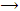Group Cations

Explain how dissolving the Group IV carbonate precipitate with 6M CH3COOH, followed by the addition of extra acetic acid, establishes a buffer with a pH of approximately

#### Related Questions in Chemistry

• ##### Q :What do you mean by the term alum What

What do you mean by the term alum? Also illustrate its uses?

• ##### Q :Explain preparation and properties of

It may be prepared by the action of phosphorus on thionyl chloride.

P4 + 8SOCl24

• ##### Q :Various cons of eating the organic foods

Describe the various cons of eating the organic foods? Briefly illustrate it.

• ##### Q :Means of molal solution Choose the

Choose the right answer from following. A molal solution is one that contains one mole of a solute in: (a) 1000 gm of the solvent (b) One litre of the solvent (c) One litre of the solution (d) 22.4 litres of the solution

• ##### Q :Normality of solution containing

Can someone please help me in getting through this problem. Determine the normality of a solution having 4.9 gm H3PO4 dissolved in 500 ml water: (a) 0.3  (b) 1.0  (c) 3.0   (d) 0.1

• ##### Q :How to calculate solutions ionic

Transference numbers and molar conductors can be used to calculate ionic mobilities.

This tables under is giving the transference numbers for positive ions at 25 degree C and the values obtained by extrapolation to infinite dilution:

[Image_Link]http:

• ##### Q :Relative lowering of vapour pressure

Which of the following solutions will have a lower vapour pressure and why? a) A 5% aqueous solution of cane sugar. b) A 5% aqueous solution of urea.

• ##### Q :Dipole attractions for london dispersion

Illustrate how are dipole attractions London dispersion forces and hydrogen bonding similar?

• ##### Q :Vapour pressure Vapour pressure of

Vapour pressure of methanol in water Give me answer of this question. An aqueous solution of methanol in water has vapour pressure: (a) Equal to that of water (b) Equal to that of methanol (c) More than that of water (d) Less than that of water